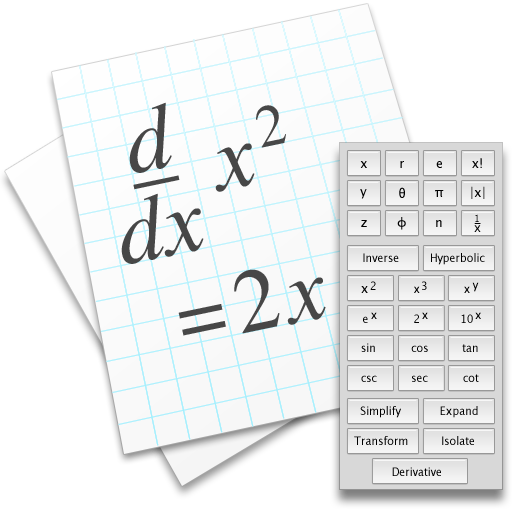Equation Calculator
1.1

5.0# Equation Calculator

1.113 October 2011

Scientific calculator with algebra, calculus and numeric computation.\$3.99
13 October 2011

5.0

See all reviews (1)

Category:

Finance

Developer Website:# Equation Calculator

1.113 October 2011

Scientific calculator with algebra, calculus and numeric computation.

## Overview

Equation Calculator is a scientific calculator which does symbolic and algebraic manipulation, algebra and calculus as well as numeric computation. You can define variables and functions, evaluate symbolic derivatives, numeric integrals and matrix operations.

### What's new in Equation Calculator

Version 1.1:
• Users can now type text comments in documents.

### Requirements for Equation Calculator

• Mac OS X 10.6.8 or later

## 1 Reviews

Rate this app:05 January 2017

Like
Version 1.105 January 2017
Like
Version 1.1
\$3.99

5.0

Category:

Finance

Developer Website:

\$3.99

5.0

Category:

Finance

Developer Website:

Don't like new MacUpdate? Back to old design.

## 1 Reviews

Rate this app:05 January 2017